Courses

# Practice Test: Ratio & Proportion- 2

## 10 Questions MCQ Test Quantitative Aptitude for Banking Preparation | Practice Test: Ratio & Proportion- 2

Description
This mock test of Practice Test: Ratio & Proportion- 2 for Quant helps you for every Quant entrance exam. This contains 10 Multiple Choice Questions for Quant Practice Test: Ratio & Proportion- 2 (mcq) to study with solutions a complete question bank. The solved questions answers in this Practice Test: Ratio & Proportion- 2 quiz give you a good mix of easy questions and tough questions. Quant students definitely take this Practice Test: Ratio & Proportion- 2 exercise for a better result in the exam. You can find other Practice Test: Ratio & Proportion- 2 extra questions, long questions & short questions for Quant on EduRev as well by searching above.
QUESTION: 1

### The ratio of the number of boys and girls in a college is 7 : 8. If the percentage increase in the number of boys and girls be 20% and 10% respectively, what will be the new ratio?

Solution: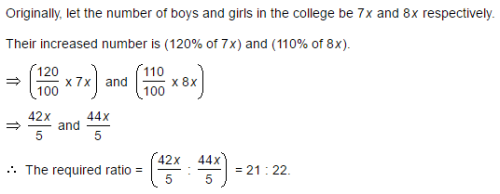QUESTION: 2

### Salaries of Ravi and Sumit are in the ratio 2 : 3. If the 'salary of each' one of them is increased by Rs. 4000, the new ratio becomes 40 : 57. What is Sumit's salary?

Solution: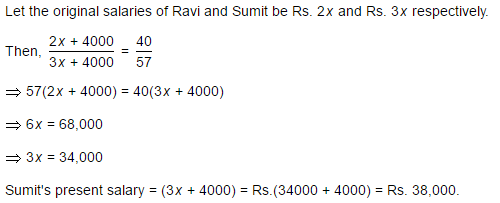QUESTION: 3

### The salaries A, B, C are in the ratio 2 : 3 : 5. If the increments of 15%, 10% and 20% are allowed respectively in their salaries, then what will be new ratio of their salaries?

Solution: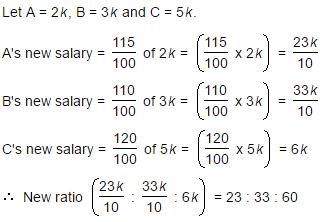QUESTION: 4

If 40% of a number is equal to two-third of another number, what is the ratio of first number to the second number?

Solution: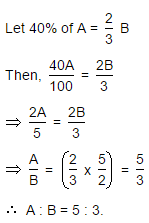QUESTION: 5

The fourth proportional to 5, 8, 15 is:

Solution: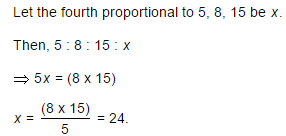QUESTION: 6

In a bag, there are coins of 25 p, 10 p and 5 p in the ratio of 1 : 2 : 3. If there is Rs. 30 in all, how many 5 p coins are there?

Solution: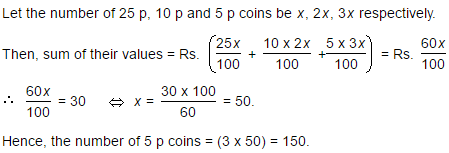QUESTION: 7

Two number are in the ratio 3 : 5. If 9 is subtracted from each number, the new numbers are in the ratio 12 : 23. The smaller number is:

Solution: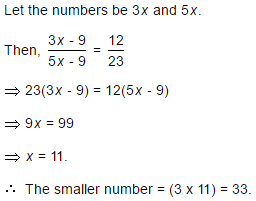QUESTION: 8

If 0.75 : x :: 5 : 8, then x is equal to:

Solution: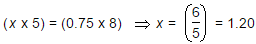QUESTION: 9

The sum of three numbers is 98. If the ratio of the first to second is 2 :3 and that of the second to the third is 5 : 8, then the second number is:

Solution: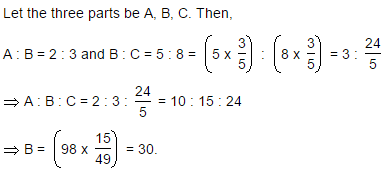QUESTION: 10

In a garrison of 3600 men, the provisions were sufficient for 20 days at the rate of 1.5 kg per man per day. If x more men joined, the provisions would be sufficient for 12 days at the rate of 2 kg per man per day. Find x.

Solution:

Let x be the number of new men joined the garrison,
The total quantity of food is = 3600(20) (1.5) kg ----------1
Now the available food will be consumed by (3600 + x) men
(3600 + x) (12) (2) kg  --------------2
1 = 2
Solving both the equations
3600(20) (1.5) = (3600 + x) (12) (2)
108000 = 86400 + 24x
21600 = 24x
X = 900
900 more men joined the garrison.